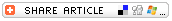#Magic SumStudents worked hard all week? Do they deserve a special reward for a job well done? This activity will offer students a nice break from structured learning. Adapt it to fit your needs. Have some Friday Fun!

## DESCRIPTION

• Have students draw five boxes in a column on their papers.
• Ask each student to pick a 2-digit number and write that number in the first box on their papers.
• Tell students you will predict their magic sums just from knowing the number in the first box on their papers -- before any other numbers are chosen. You can do that by asking each student to tell you the number he or she wrote in the first box. Record each student's name and number. To find each student's magic sum, add 198 to the number they wrote in the first box. (Find the number more quickly by adding 200, then subtracting 2.) Record those sums on your paper, and put the paper out of your reach.
• Ask a student to pick any 2-digit number. Have all students write the number that student called out in the second box on their papers.
• Tell students that you will pick the number for the third box on their papers. (To choose a number for that box, subtract the number the student provided for the second box from 99.) Pretend to choose a number at random and have all the students write your number in the third box.
• Ask another student for a 2-digit number, and have all students write that number in the fourth box on their papers.
• Tell students that you will pick a 2-digit number for the fifth box. (To arrive that number, subtract the number the student called out for the fourth box from 99.) Pretend to pick a number at random, and have students write that number in the fifth box.
• Ask students to add the numbers in their boxes to find the magic sum.
• Display your "mathemagic" powers by having a student read the name of each student and the magic sum you predicted for that student.

By now, students might suspect that there is some kind of "trick" or "special math" involved in this activity that enabled you to get all the Magic Sums correct. Challenge them to figure out the trick.

Click the link below for a simple explanation of the magic behind this Magic Sum activity.